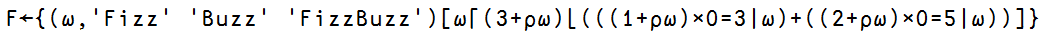# APL – solving Fizz Buzz

Note: see the rest of the series so far.

This is a classic interview question, and after some experimentation I ended up with the following solution in APL:$latex F \ \iota 100$

=> 1 2 Fizz 4 Buzz Fizz 7 8 Fizz Buzz 11 Fizz 13 14 FizzBuzz 16 17 Fizz 19 Buzz Fizz 22 23 Fizz Buzz 26 Fizz 28 29 FizzBuzz 31 32 Fizz 34 Buzz Fizz 37 38 Fizz Buzz 41 Fizz 43 44 FizzBuzz 46 47 Fizz 49 Buzz Fizz 52 53 Fizz Buzz 56 Fizz 58 59 FizzBuzz 61 62 Fizz 64 Buzz Fizz 67 68 Fizz Buzz 71 Fizz 73 74 FizzBuzz 76 77 Fizz 79 Buzz Fizz 82 83 Fizz Buzz 86 Fizz 88 89 FizzBuzz 91 92 Fizz 94 Buzz Fizz 97 98 Fizz Buzz

The answer looks correct, though this is perhaps not the most straight forward solution, so let’s walk through what’s happening here:

• first turn the input array $latex \omega$ into booleans to determine if they’re divisible by 3 $latex 0=3 | \omega$ or 5 $latex 0=5 | \omega$

$latex 0 = 3 | \omega$ : 0 0 1 0 0 1 0 0 1 0 0 1 …

$latex 0 = 5 | \omega$ : 0 0 0 0 1 0 0 0 0 1 0 0 …

• assuming that $latex \omega$ has length of 100, then transform the boolean arrays from the previous step so that 1s becomes 101s (for those divisible by 3) and 102s (for those divisible by 5)

$latex (1 + \rho \omega) \times 0 = 3 | \omega$ : 0 0 101 0 0 101 0 0 101 0 0 101…

$latex (2 + \rho \omega) \times 0 = 5 | \omega$ : 0 0 0 0 102 0 0 0 0 102 0 0 0…

• add the two arrays together, so that those divisible by 3 has value 101, divisible by 5 has value 102 and those divisible by 15 has value 203

0 0 101 0 102 101 0 0 101 102 0 101 0 0 203 0 0 101 …

• apply minimum $latex \lfloor$ to this array with 103 being the operand, so now numbers divisible by 15 has value 103 instead of 203

0 0 101 0 102 101 0 0 101 102 0 101 0 0 103 0 0 101 …

• apply maximum $latex \lceil$ to this array with the input array $latex \omega$ to end up with the following

1 2 101 4 102 101 7 8 101 102 11 101 13 14 103 16 17 101

• use this array as index for an array that is made up of the input array $latex \omega$ with ‘Fizz’ ‘Buzz’ ‘FizzBuzz’ added to the end at index position 101, 102 and 103 respectively

### 3 thoughts on “APL – solving Fizz Buzz”

1.Randy A MacDonald

My take:

F?{? ‘Buzz’ ‘Fizz’ ‘FizzBuzz’??3 2 1 0?2?×3 5|?}
15? F¨?100
1 2 Fizz 4 Buzz Fizz 7 8 Fizz Buzz 11 Fizz 13 14 FizzBuzz

since the introduction of each (¨) in the 80s, the need to process entire arrays for what is essentially an item-by-item problem is of academic or enthusiast interest. The array capabilities of APL can still be showcased: 3|x and 5|x can be combined into 3 5|x, for example, and encode(?) can convert a two bit list into an integer

2.Thanks for sharing :-)

3.Dyalog publishes a one-liner each week on #onelinerwednesday with APL one-line; the FizzBuzz one-liner is:

{?(3?(0=3 5|?)?1)/’Fizz’ ‘Buzz’?}¨?20